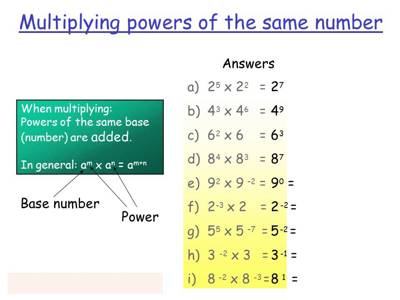Powers of Multiplication or Exponents

We know how to calculate the expression 5 x 5. This expression can be written in a shorter way using something called exponents.

5⋅5 = 52

An expression that represents repeated multiplication of the same factor is called a power.

The number 5 is called the base, and the number 2 is called the exponent. The exponent corresponds to the number of times the base is used as a factor.

Raising to powers by repeated multiplication

The result of raising a number (the base) to a power (the exponent) is the same number that would be obtained by multiplying the base number together with the number of times that is equal to the exponent.

Example: 34 = 81 is equivalent to 3*3*3*3 = 81.

In this example, the base is 3 and the exponent is 4.

Exponents are written as a superscript number (e.g. 34) or preceded by the caret (^) symbol (e.g. 3^4).Explanation:

The “exponent”, being 3 in this example, stands for however many times the value is being multiplied. The thing that’s being multiplied, being 5 in this example, is called the “base”.

This process of using exponents is called “raising to a power”, where the exponent is the “power”. The expression “53” is pronounced as “five, raised to the third power” or “five to the third”.

The thing that’s being multiplied, being 5 in this example, is called the “base”. This process of using exponents is called “raising to a power”, where the exponent is the “power”.

First, any number raised to the power of “one” equals itself. This makes sense because the power shows how many times the base is multiplied by itself. If it’s only multiplied one time, then it’s logical that it equals itself.

Secondly, one raised to any power is one. This, too, is logical, because one time one time one, as many times as you multiply it, is always equal to one.

Information Source: